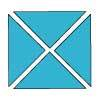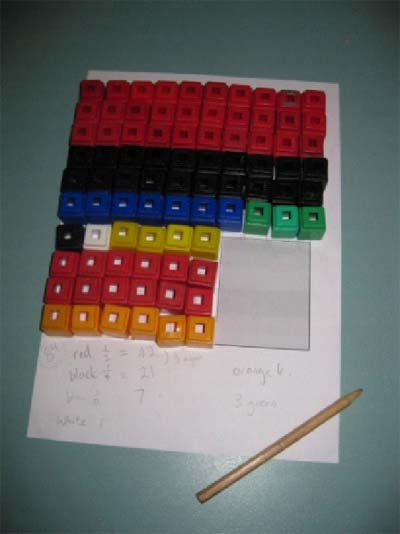#### You may also like### Chocolate

There are three tables in a room with blocks of chocolate on each. Where would be the best place for each child in the class to sit if they came in one at a time?### Four Triangles Puzzle

Cut four triangles from a square as shown in the picture. How many different shapes can you make by fitting the four triangles back together?### Cut it Out

Can you dissect an equilateral triangle into 6 smaller ones? What number of smaller equilateral triangles is it NOT possible to dissect a larger equilateral triangle into?

# Fractions in a Box

##### Age 7 to 11Challenge Level

This was a tricky problem. Well done to those of you who had a go. We had some very clearly explained answers. The key was to work out the size of the booklet first.

Rachel, Ol, Jack and Alex from Moretonhampstead Primary said:

First we worked out how many squares the booklet is. The number has to be a square number and has to be even and $\frac{1}{4}$ of that number has to be even again. The only number possible for that is $1$6 (four squared).
Then we took $16$ (that was how big the booklet was) from a $100$ which is $84$ (there are $84$ squares to play the game with). With $84$ we can answer the first question - how many discs are there altogether? ($84$).
After that we worked out how many discs there would be for the colours. We worked out there would be $42$ discs ($\frac{1}{2}$ of $84$), $21$ black discs ($\frac{1}{4}$ of $84$) and $7$ blue ($\frac{1}{12}$ of $84$).
We put that on the grid as it says on the sheet. Next we used the last full column for blue and green. We know that there are $7$ blues (because of what we worked out earlier) which means there are $3$ green discs ($7 +3 =10$ (which is how many in a column)).
There were five squares left. It says that there is $1$ white square then the leftovers are yellow so we had $4$ yellows and $1$ white disc.
Now we hade completed the grid we could answer question $2$ and $3$. For question $2$ we counted up all the orange squares ($6$) and the fraction is $\frac{6}{84}$ but we had to simplify it to $\frac{1}{14}$ (the answer).
Lastly we did question $3$. The fraction of green is $\frac{3}{84}$ and simplified for the actual answer is $\frac{1}{28}$.
The fraction of yellow is $\frac{4}{84}$ and simplified for the answer is $\frac{1}{21}$.
There is only one white square so the answer is $\frac{1}{84}$, for white.

Hamish, Rory, Sarah, Jesse and Samuel from Rutherglen Primary also reasoned very clearly and they sent us a picture of the full box which they modelled using cubes:Sophie and Claire from The Downes School wrote:

$1\times 1$ didn't work because it said that two shortened rows have red discs.
$2\times 2$ didn't work because you need two shortened rows of red and one of orange.
$3\times 3$ didn't work because the total number of discs would be odd and you couldn't halve it. This means all odd numbers didn't work.
$4\times 4$ did work because you had the right amount of shortened rows.
$6 \times 6$ didn't work because you can't divide $64$ by $12$.
$8 \times 8$ didn't work because you need six whole rows.

Emma, Abi, Matthew B and Yuji from Moorfield Junior School; Keshinie and Sharon at Kilvington GGS Victoria, Australia; Gideon from Newberries Primary School and Hannah, Georgia, Patrick; Hana from Bali International School and Matthew from Brighton College Prep School realised that the number left after taking away the booklet must be a multiple of $12$. Keshinie and Sharon describe how they continued from there:

So that made it $84$.
Half of the disks are red so that made the amount of red $42$.
Then it said that a quarter is black so that made it $21$.
Then it said that one twelfth is blue so that made it $7$.
Then it said that one complete row was filled with all of blue and green and the remainder of $10$ if you take away $7$ made it $3$ green.
Then it said that one of the shortened rows is exactly filled with all the orange disks so that makes it $6$.
Then it said that there was only one white disk.
Then we added all the numbers together making $80$ disks so there was a remainder of $4$ which had to be yellow.

We divided the $84$ disks by the $6$ orange ones that made it $14$. So the fraction of orange had to be $1$ out of $14$ ($\frac{1}{14}$).
We divided the $84$ disks by the $3$ green disks making the answer $28$. So the fraction of green had to be $\frac{1}{28}$.
We already knew that the fraction of white disk was $\frac{1}{84}$.
We divided the $84$ disks by the $4$ yellow ones making it $21$ so the fraction of yellow had to be $\frac{1}{21}$.

James from the Charter School explained very well how he went about the problem:

Each of the sides is $10$ units and I called each of the sides of the booklet $x$. this means that the equation for finding the number of discs was $N=100-x^2$. ($N$ being the number of remaining discs).
The amount of Blue discs was $\frac{N}{12}$ meaning that $N$ was a multiple of $12$. So I then collected all of the multiples of $12$: $12$, $24$, $36$, $48$, $60$, $72$, $84$, $96$. I then eliminated those that did not fit the earlier equation because there was not a square number that fitted. This left: $36$, $84$, $96$.
I eliminated $96$ because $x$ had to be more that three for there was one complete row of orange discs and two of red discs.
This left: $36$, $84$. From this I deduced that $x$ had to be $4$ or $8$. This means that the amount of red disks had to end in a $2$ or a $4$, because there are two incomplete rows of red these either have to be a length of $6$ or $2$. Since the amount of red discs is half of $N$ I halved both my possible $N$'s which came up with $18$ and $42$. This means that the amount of reds was $42$ and $N$ was $84$.
This means that $x$ is $4$ and that the amount of orange discs was $6$ meaning the fraction is $\frac{1}{14}$.
The amount of blue was $\frac{N}{12}$ which was $7$.
This means that the amount of green discs was $3$ because blues + greens = $10$. That means the fraction was $\frac{1}{28}$.
The amount of white was $1$ meaning the fraction was $\frac{1}{84}$.
Finally the rest were yellow.
Red was $42$. Blacks was $\frac{N}{4}$ which was $21$. Blue was $7$. Orange was $6$. Green was $3$. White was $1$.
If you take all those away from $84$ you end up with $4$. That is the amount of yellows. This means the fraction is $\frac{1}{21}$.

Well done too to Harriet and Harah from Greenacre School for Girls, Ruairidh from St Mary's High School, Anne-Marie, Emma, Katherine, Laura from Gorseland Primary and Eulalie and Holly who go to Lympstone Primary School.